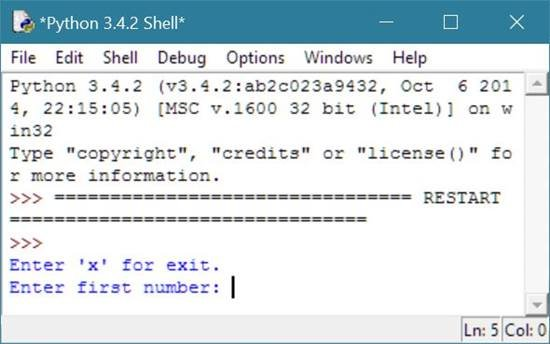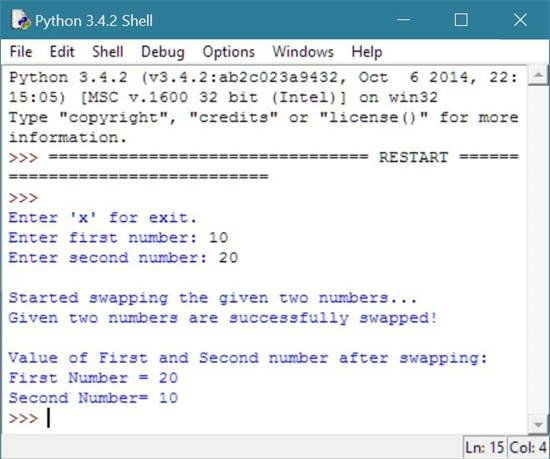# Python Program to Swap Two Numbers

## Swap Two Numbers in Python

To swap two numbers in python, you have to ask from user to enter value of two numbers to swap those two numbers using the third variable. Swapping of two number means placing the value of first variable to second variable and the value of second variable to the first variable just by using another temporary variable.

## Python Programming Code to Swap Two Numbers

Following python program will ask from the user to enter the value of two numbers to swap both the number.

To swap two numbers in python, first store the value of the first number to a temporary variable say swap, then store the value of the second variable to the first variable, and finally store the value of the temporary variable say swap to the second variable as shown in the program given below:

```# Python Program - Swap Two Numbers

print("Enter 'x' for exit.");
num1 = input("Enter first number: ");
if num1 == 'x':
exit();
else:
num2 = input("Enter second number: ");
print("\nStarted swapping the given two numbers...");
number1 = int(num1);
number2 = int(num2);
swap = number1;
number1 = number2;
number2 = swap;
print("Given two numbers are successfully swapped!\n");
print("Value of First and Second number after swapping:");
print("First Number =",number1,"\nSecond Number=",number2);
```

Here is the sample run of the above python program to show how to swap two numbers in python:Above screenshot shows the initial sample output produced after running the above program. This will ask from user to enter any two number say 10 as first number and 20 as second number to swap this two number after pressing the enter key as shown in the below sample output or run:Here is the same program with run on python shell:### Same Program in Other Languages

You may also like to learn or practice the same program in other popular programming languages:

Tools
Calculator

Quick Links
Signup - Login - Give Online Test